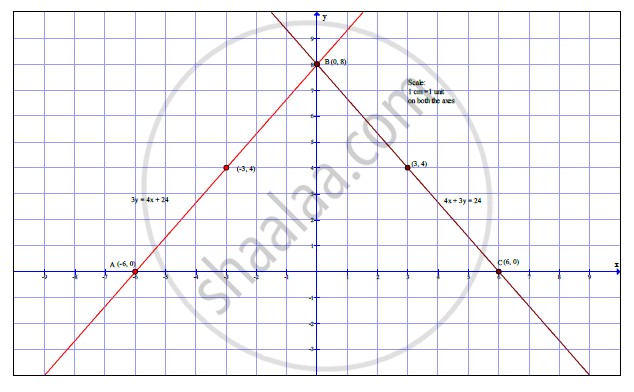# Draw the graphs representing the equations 4x + 3y = 24 and 3y=4x+24 on the same graph paper. Write the co-ordinates of the point of intersection of these lines and find the area of triangle formed by these lines and the X-axis. - Algebra

Draw the graphs representing the equations 4x + 3y = 24 and 3y=4x+24 on the same graph paper. Write the co-ordinates of the point of intersection of these lines and find the area of triangle formed by these lines and the X-axis.

#### Solution

The given simultaneous equations are 4x + 3y = 24 and 4x - 3y = -24.

4x+3y =24 …........(i)
y =(24−4x)/3

 x 0 3 6 y 8 4 0 (x,y) (0,8) (3,4) (6,0)

4x-3y =-24 …...........(ii)
y =(4𝑥+24)/3

 x 0 -3 -6 y 8 4 0 (x,y) (0,8) (-3,4) (-6,0)From the graph A (-6, 0), B(0, 8) C (6, 0) and AC = 12 units.
Height = h= 8 units
Base = b = 12 units

Area of triangle=(1/2) x Base x Height

A(ΔABC)= (1/2) x 12 x8

A(ΔABC)=48 sq units

Concept: Graphical Method of Solution of a Pair of Linear Equations
Is there an error in this question or solution?